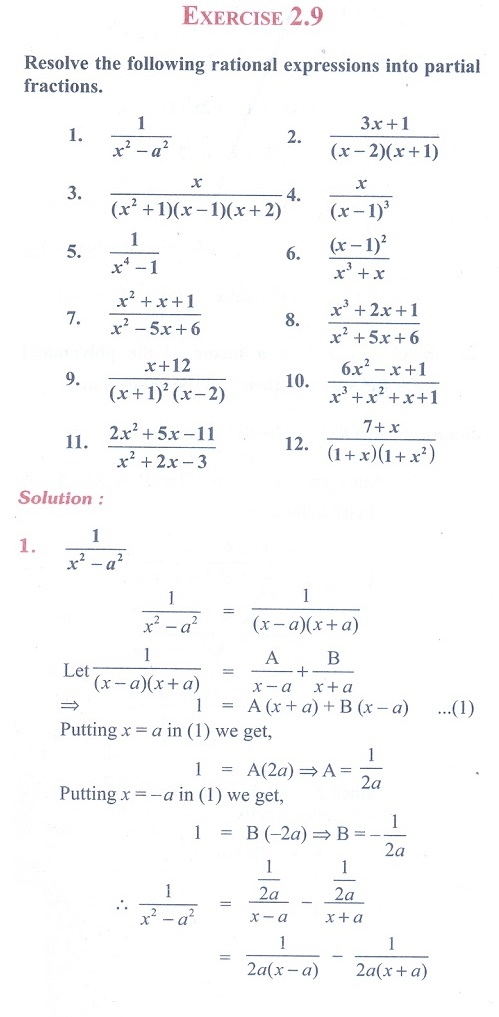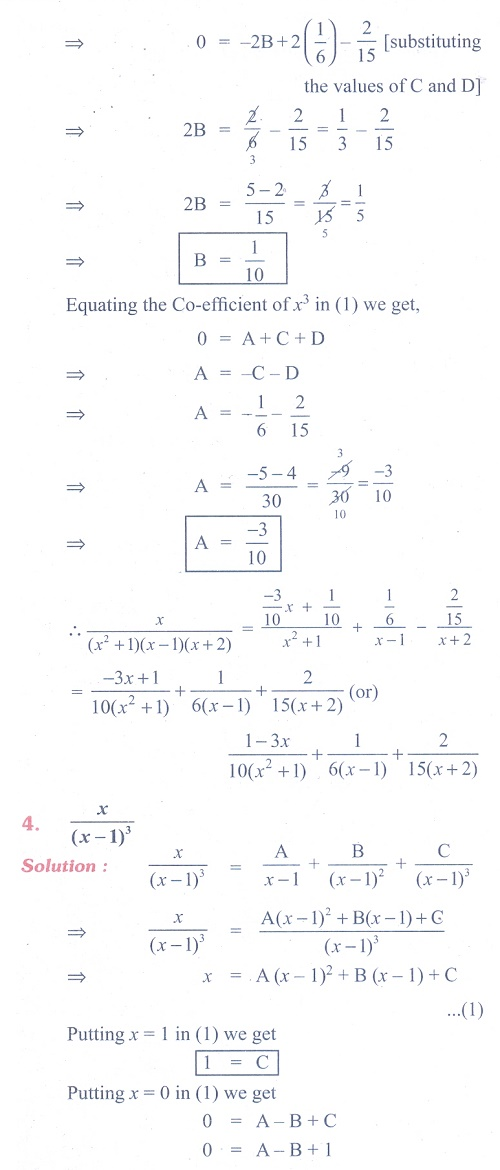Home | | Maths 11th std | Exercise 2.9: Partial Fractions

# Exercise 2.9: Partial Fractions

Maths Book back answers and solution for Exercise questions - Mathematics : Basic Algebra: Partial FractionsTags : Problem Questions with Answer, Solution | Basic Algebra | Mathematics , 11th Mathematics : UNIT 2 : Basic Algebra
Study Material, Lecturing Notes, Assignment, Reference, Wiki description explanation, brief detail
11th Mathematics : UNIT 2 : Basic Algebra : Exercise 2.9: Partial Fractions | Problem Questions with Answer, Solution | Basic Algebra | Mathematics